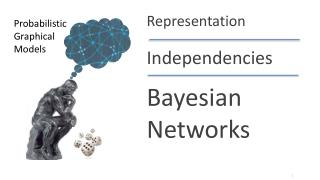Download PresentationBayesian NetworksBayesian Networks - PowerPoint PPT Presentation

Download PresentationBayesian Networks
An Image/Link below is provided (as is) to download presentation

Download Policy: Content on the Website is provided to you AS IS for your information and personal use and may not be sold / licensed / shared on other websites without getting consent from its author. While downloading, if for some reason you are not able to download a presentation, the publisher may have deleted the file from their server.

- - - - - - - - - - - - - - - - - - - - - - - - - - - E N D - - - - - - - - - - - - - - - - - - - - - - - - - - -
Presentation Transcript

1. Representation Probabilistic Graphical Models Independencies BayesianNetworks

2. Independence & Factorization • Factorization of a distribution P implies independencies that hold in P • If P factorizes over G, can we read these independencies from the structure of G? P(X,Y) = P(X) P(Y) X,Y independent • P(X,Y,Z)  1(X,Z) 2(Y,Z) (X  Y | Z)

3. Flow of influence & d-separation Definition: X and Y are d-separated in G given Z if there is no active trail in G between X and Y given Z D I G S L Notation: d-sepG(X, Y | Z)

4. Factorization  Independence: BNs Theorem: If P factorizes over G, and d-sepG(X, Y | Z) then P satisfies (X  Y | Z) D I G S L

5. Any node is d-separated from its non-descendants given its parents Coherence Difficulty Intelligence If P factorizes over G, then in P, any variable is independent of its non-descendants given its parents Grade SAT Letter Job Happy

6. I-maps • d-separation in G  P satisfies corresponding independence statement • Definition: If P satisfies I(G), we say that G is an I-map (independency map) of P I(G) = {(X  Y | Z) : d-sepG(X, Y | Z)}

7. I-maps P2 P1 G1 G2 D I D I

8. Factorization  Independence: BNs Theorem: If P factorizes over G, then G is an I-map for P

9. Independence  Factorization Theorem: If G is an I-map for P, then P factorizes over G D I G S L

10. Summary Two equivalent views of graph structure: • Factorization: G allows P to be represented • I-map: Independencies encoded by G hold in P If P factorizes over a graph G, we can read from the graph independencies that must hold in P (an independency map)